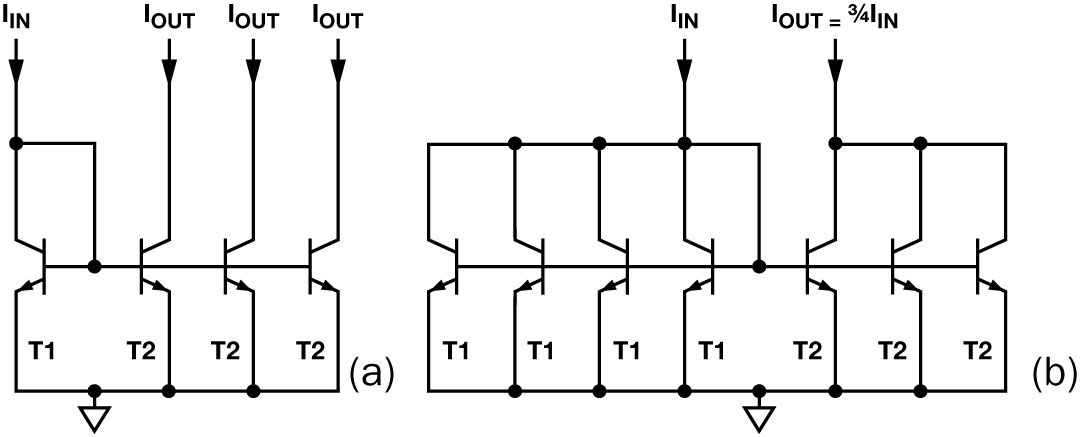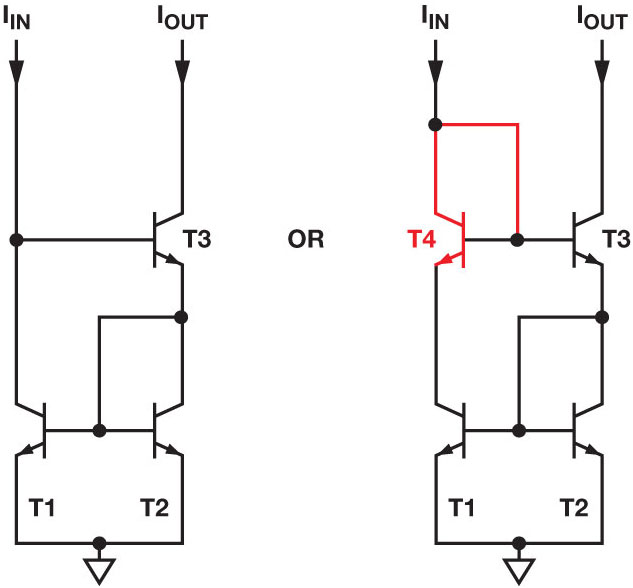# Current-Output Circuit Techniques Add Versatility to Your Analog Toolbox

Although current mirrors and circuits such as the Howland current source are taught in analog circuit courses, a surprising number of engineers tend to think exclusively in terms of voltage when defining the output of precision analog circuitry. This is a pity, as current outputs offer advantages in a number of situations, including analog current-loop signaling (0 mA to 20 mA and 4 mA to 20 mA) in high-noise environments, and level shifting an analog signal across a large potential difference without the use of optical or magnetic isolation techniques. This article summarizes some of the techniques available and suggests a number of useful circuits.

It is remarkably easy to obtain a steady current output. The simplest method uses a current mirror: two identical transistors—manufactured on the same chip so that their process, geometry, and temperatures are all identical—are connected as shown in Figure 1. The base-emitter voltage is the same for both devices, so the output current flowing in the collector of T2 is the same as the input current flowing in the collector of T1.

This analysis assumes that T1 and T2 are identical and isothermal, and that their current gain is so large that the base currents can be disregarded. It also ignores the Early voltage, which causes the collector current to vary with varying collector voltage.

These current mirrors can be made with NPN or PNP transistors. By forming T2 with n transistors connected in parallel, the output current will be n times the input current, as shown in Figure 2a. If T1 consists of m transistors and T2 of n transistors, then the output current will be n/m times the input current, as shown in Figure 2b.Figure 2. (a) Multistage current mirror, and (b) noninteger-ratio current mirror. The three T2 collectors may be joined to give 3IIN.

If the effect of Early voltage is important, it can be minimized by using the slightly more complex Wilson current mirror. Both three-transistor and four-transistor versions are shown in Figure 3. The four-transistor version is more accurate and has a wider dynamic range.Figure 3. Wilson current mirror. T4 is optional but improves accuracy and dynamic range.

When a transconductance amplifier (voltage_in/current_out) is required, it can be made with a single-supply op amp, a BJT or FET (a MOSFET is usually the best choice as there is no base current error), and a precision resistor that defines the transconductance, as shown in Figure 4.

This circuit is both simple and inexpensive. The voltage on the MOSFET gate sets the current in the MOSFET and R1 such that V1, the voltage across R1, equals the input voltage, VIN.

If a current mirror is required inside a monolithic IC, the simple transistor current mirrors are ideal. With discrete circuitry, however, the high price of matched transistors (due to their limited demand, rather than any manufacturing difficulties) makes the op-amp current mirror shown in Figure 5 the least expensive technique. This current mirror uses a transconductance amplifier plus one additional resistor.

Current mirrors have relatively high, sometimes nonlinear input impedance, so they must be fed by a current from a high-impedance current source (sometimes known as a stiff current source). An op amp is required if the input current must have a low-impedance sink. Figure 6 shows two low ZIN current mirrors.Figure 6. (a) Inverting low ZIN current mirror, and (b) noninverting low ZIN current mirror.

With basic current mirrors and sources, the input and output current polarities are the same. Usually the emitters/sources of the output transistors are grounded, directly or through a sense resistor, and the output current flows from the collector/drain to a load, the other terminal of which is connected to a dc supply. This is not always convenient, especially when one terminal of the load must be grounded. This is not a problem if the circuit may be built with its emitter/source on the dc supply, as shown in Figure 7.

If the current or voltage input is referred to ground, level shifting must be used. Various circuits are possible, but the system shown in Figure 8 is suitable in many situations. This simple circuit uses a current source on ground to drive a current mirror on the dc supply, which drives the load. Note that the current mirror may have gain, so the signal current need not be as large as the load current.

The circuits we have discussed so far are unipolar—the current flows in one direction—but it is also possible to make bipolar current circuits. The simplest and best known is the Howland current pump, illustrated in Figure 9. This simple circuit has a number of problems: it requires very precise resistor matching to obtain high output impedance; the input source impedance adds to the resistance of R1, so it must be very low to minimize the matching error; the supply voltages must be substantially higher than the maximum output voltage; and the CMRR of the op amp must be reasonably good.

Today's high-performance instrumentation amplifiers (in-amps) are not expensive, so it is quite simple to make a bipolar current source using an op amp, an in-amp, and a current-sense resistor, as shown in Figure 10. Such circuits are simpler than the Howland pump, are not dependent on a resistor network (except the one integrated with the in-amp), and may have a voltage swing to within about 500 mV of each supply.

The circuits we have considered up to this point are amplifiers with precision current outputs. They may, of course, be used with a fixed input as accurate current sources, but it is possible to build simpler 2-terminal current sources. The ADR291 low-current voltage reference has about 10 μA standby current with a 20 nA/°C typical temperature coefficient. Adding a load resistance as shown in Figure 11, the reference current over a 3-V to 15-V supply range is (2.5/R + 0.01) mA, where R is the load resistance in kΩ.

If precision is not an issue, and all that is required is a stiff unipolar current source, a current source can be built with a depletion- mode JFET and a resistor. This arrangement, shown in Figure 12, is not particularly temperature stable and, for a given value of R, the current can vary considerably from device to device, but it is simple and inexpensive.

I recently needed power for some LEDs. Several engineer friends thought that I would have trouble making the variable current supply that is required for them to be dimmed. In fact, I quickly modified some "black-brick" laptop power supplies (bought for pennies at a car boot sale) to do the job. Figure 13 shows a simple modification that supplies constant current to the LEDs. With small output currents, it operates normally with a fixed-output voltage.Figure 13. Black-brick switching supply modified for current-limit output.

To make a variable current, a voltage reference—from the black brick or local—is applied to a potentiometer represented by P1 and P2. OPA2 and the MOSFET send a small current through R1, causing a voltage drop across it. The load current flows in the sense resistor. If the voltage drop in the sense resistor due to the load current exceeds the drop across R1, the output of OPA1 will rise, overriding the voltage control in the brick, and limiting its output voltage to prevent the output current from exceeding the limit.

This discussion of basic current-source ideas is not a detailed application note. Some of the circuits require further design work to limit (or dissipate) heat, to ensure amplifier stability and that no absolute-maximum ratings are exceeded, and to calculate practicable performance limits. For more detailed analyses of these circuits, consult a good electronics textbook, the Analog Devices website, or even Wikipedia.

### Ссылки

AN-1208 Application Note. Programmable Bidirectional Current Source Using the AD5292 Digital Potentiometer and the ADA4091-4 Op Amp. Analog Devices, Inc., 2013.

Murnane, Martin. AN-968 Application Note. Current Sources: Options and Circuits. Analog Devices, Inc., 2008.